Combo Offer 35% Off + 10% Extra OFF on WhatsApp
Geotechnical Engineering: Design And Plaxis Modelling Challenge
Get Upto 50% off

## Geotechnical Engineering: Design And Plaxis Modelling Challenge

Executive Summary

In this assignment, the design of the RC-L-shaped cantilever and the strip foundation is described briefly with proper justification. The analysis of the design is based on the different failure mechanism techniques that are mentioned in the assignment. It has been observed that the cantilever system is performed in different conditions under the parameters of the failure mechanism. The design of the model is done by using the PLAXIS simulation technique. The mathematical expressions for the bearing capacity are discussed in this assignment. The cantilever retaining wall is designed according to assumptions that are considered while designing the overall system. An axis-symmetric model of Single pile problem definition has been assessed with the PLAXIS software solutions. Shallow raft foundation problem definition has also been implied with the calculation of un-drained and drained cohesion.

Get free samples written by our Top-Notch subject experts for taking online assignment help & australian assignment help services.

# Question 1

## 1.1 Problem Definition

The first question is regarding the design of the RC-L-shaped cantilever with different optimum conditions. The cantilever is a structural element that is designed horizontally and is supported in one end. The cantilever retaining wall is shown in the assignment. As commented by YAVAN et al. (2020, p.552), the main task of this question is to design a suitable cantilever that satisfies the failure mechanisms such as overturning, sliding, and bearing capacity. Hence, some numerical calculations are performed to develop the effect of the failure mechanism parameters. Besides, the concept of wall material, interface, and foundation slab are described briefly in this section. Different type of design parameters is introduced to design the cantilever with the assumptions. An excel sheet is developed in order to record the calculations for the design of the cantilever. The justification of the optimum design of RC-L-shaped cantilever is done in this question.

## 1.2 Problem analysis

The analysis of this design process is performed using different numerical solutions. The optimum design is based on the bearing capacity, overturning, and sliding parameters. The design is developed in such a way that it should maximize the benefits with less use of materials (Yadav et al. 2021, p.230). The bearing capacity is defined as the horizontal pressure on the backfill side which is used to generate the overturning in the base. When the electricity supply is low the bearing pressure is the maximum under the compression of footing.

The formula for bearing capacity is,

Bearing capacity= Rv / (0.75 * L – 1.5 * e)

L is the length of the cantilever and Rv is the vertical force inside the cantilever.

The overturning is the moment that is associated with the axial load which is placed in the RC-L-shaped cantilever.

The formula of the overturning moment is,

Overturning moment= ? Horizontal forces with respect to the distance of the footing base

The formula of sliding is,

Sliding = (resisting force/driving lateral force)

The analysis of this design is based on the above formulas.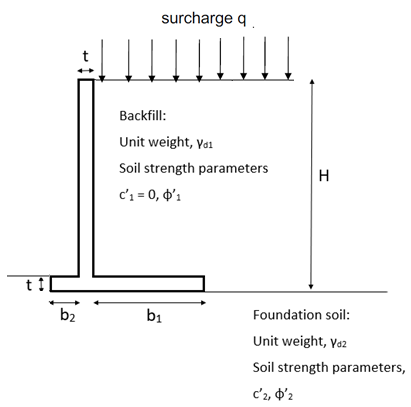Figure 1.1: Diagram of Cantilever retaining wall

(Source: Self Created)

## 1.3 Results and discussion

The results are obtained by using different formulas of the parameters. The optimum geometrical design is developed with appropriate length in the foundation slab. The design of the cantilever system is designed in such a way that the overall system should maintain the failure mechanisms such as overturning, sliding, and bearing capacity. As stated by Uray et al. (2019, p.112), the numerical calculations are performed for each of the parameters which depend on the overall mechanism of the cantilever system. On the other hand, the design of the cantilever depends on the economical aspects as the developed design should maximize the profit with less use of the components. Therefore, it has been observed that the RC-L-shaped cantilever is designed to manage the dry, cohesion less backfill parameters which are important in the retaining wall problem. The readers would be able to collect practical information regarding the different parameters of the cantilever system.

## 1.4 Conclusion

In this section, the design of the RC-L-shaped cantilever is described with practical calculations. The concept of the different parameters is discussed in this section. The three different failure mechanisms are discussed in this section. Overturning, sliding, and the bearing capacity of a cantilever are described with mathematical expression and practical considerations. The profit of the cantilever system is considered as the main parameter while designing the RC-L-shaped cantilever. The different assumptions for the cantilever are mentioned in order to design the retaining wall of the cantilever properly with better requirements. There is a part where the model is developed with different friction angles, wall materials, and foundation soil materials. The interface property is analyzed to choose different foundation soil values through which the design is developed.

# Question 2

## 2.1 Problem Definition

The model strip foundation is designed according to the requirements for the failure mechanisms. The numerical calculation of the bearing capacity is performed for different cases such as under drained loading with under drained cohesion, detained loading with drained cohesion, and increase value of friction. As commented by Chanmee et al. (2016, p.1077), the comparison of the results is done on the basis of PLAXIS calculation and theoretical calculations. This comparison is described using a single graph that represents the friction angle of the foundation soil. The results for the bearing capacity are displayed using graphical representation which is obtained from the PLAXIS simulation. Therefore, the design of the model strip foundation is designed based on the above conditions. The same analysis of the system is performed by increasing the foundation level of the strip. Again the comparison is done based on the results.

## 2.2 Problem analysis

In this section, the different numerical calculations are performed for different cases under the failure mechanisms. The foundation system is developed using these parameters.

Case1:

Bearing capacity is the amount of bearing stress which occurs in the strip foundation. When the stress of the foundation level is increased the cohesion conditions are,

qn=q-q0

q0=γ*D

D is the foundation depth and γ is the unit weight of the soil

According to Alander et al. (2020, p.2019), the formula for bearing capacity is,

qf =c.Nc +qo.Nq + ½g.B .Ng

For un-drained loading, calculations are in terms of total stresses; the un-drained shear strength (su); Nq = 1.0 and Ng = 0

c = apparent cohesion intercept

qo = g . D (i.e. density x depth)

D = founding depth

g = unit weight of the soil removed.

Case2:

For drained loading conditions, the bearing capacity is based on the stress on the strip foundation. The formula of bearing capacity is,

qf =c.Nc +qo.Nq + ½g.B .Ng

For drained loading, calculations are in terms of effective stresses; f´ is > 0 and N c, Nq, and Ng are all > 0.

qf= 1.8 (Nq - 1) tanΦ

=0.317 m^-2

qf= 1.8 (Nq - 1) tanΦ

=0.655 m^-2

qf= 1.8 (Nq - 1) tanΦ

=1.039 m^-2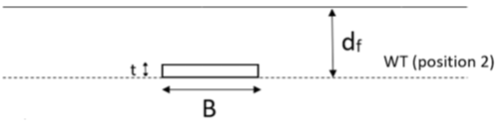Figure 2.1: Diagram of Shallow raft foundation

(Source: Self Created)

## 2.3 PLAXIS modelling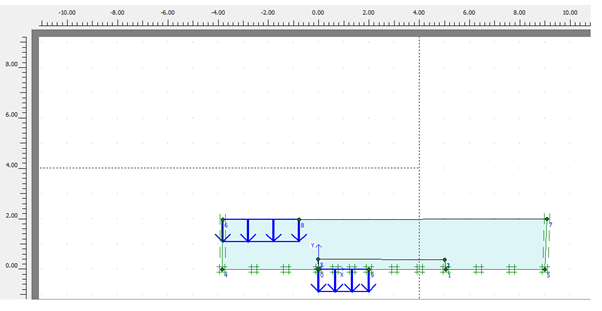Figure 2.2: PLAXIS Design

(Source: Self-created)

The diagram has been developed using the PLAXIS software and this defines the effective system measures in the software design tools. This design has effectively derived the construction of the Shallow raft foundation and these are effective in terms of analysing the structure with different materials (Lam, 2018, p.181). A design is developed according to the design specifications and these are effective in terms of deriving the idea on the system performance. These can be relevant to the implications of the system assessments and the following results have been developed that provides effectiveness for configurations. The configurations are useful to derive the relevant data from the system.

## 2.4 Results & Discussion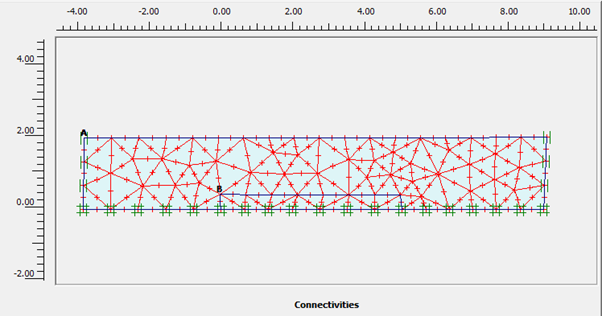Figure 2.3: PLAXIS Output

(Source: Self-created)

The output is developed based on the configuration of the system and these are subjective and relevant with the implications of the system model. These assessments are subjective in terms of determining a difficult approach for the system. An effective system is developed based on the materials of construction and these assessments are necessary for better construction of the system. The output is also effective with the objectives of the system designs and the relevance of the system is also implied with the implications of the system structure. The results are hence based on several implications and these results output on the results.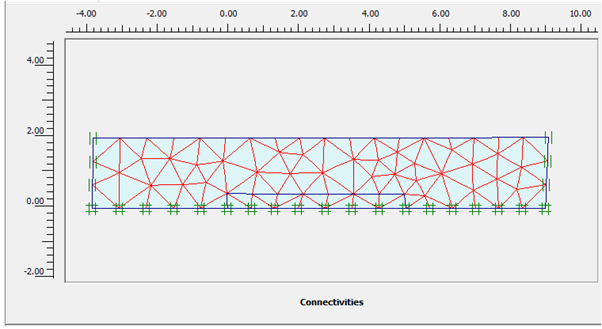Figure 2.4: PLAXIS Output

(Source: Self-created)

The report of the construction has been depicted in terms of determining the ideas on the developed construction. These constructions are effective in determining the issues based on the system development and the approaches can be rationalized with the design of the beam system and the reports on the materials also shown an effective result (Som, 2017, p.79). Thus, an effective system construction is possible and these can be relevant in terms of developing a suitable system and its performance. These can also be relative to the objections and implications of the systems and the rational development of the system is possible. The designs are hence effective with the provided material.

## 2.5 Conclusion

In this section, the design of the model strip foundation is developed under different conditions. The numerical analysis of the bearing capacity is performed according to the ultimate conditions that are mentioned in the question. Some graphical representation is done based on the analysis of the loading conditions. Therefore, the readers would be able to achieve detailed information regarding the ultimate bearing capacity and its applications. The calculation of bearing capacity, friction angle, and other parameters are done properly with mathematical expressions to simplify the analysis of the design process. PLAXIS simulation is used to design the proposed design to demonstrate the operation of the system.

# Question 3

## 3.1 Problem definition

The problem is based on the axisymmetric model of the provided structure and this is a vertical single pile problem. The problem is defined as the reinforced concrete pile and the pile can be subjective with the several cases. These cases include undrained loading indulged with the values of cu and φ = 0 and also the separate conditions with the values of drained loading as drained cohesion, c’ and friction angle, φ’ = 10, 20, and 30 degrees. The prime motive of the problem assessment and its solution can be infiltrated with the objectives of deriving ultimate bearing capacities (Zhao and You, 2018, p.11). This is to be determined with the graphical development of the factors concerning the soil friction angle, φ’. These aspects are effective in terms of determining the drained and undrained coercion and these can also be subjected to determine PLAXIS simulations based on the derived and assessed data from the provided tables.

## 3.2 Problem analysis

The formula for cohesion can be derived from the assessments as s = c + σ tan φ and these aspects have provided several variables as s = shear stress, c = cohesion, σ = normal stress, and φ = angle of friction. This is assessed from the system that an effective measure of the system is possible. These assessments are subjective to determine the relevance of the PLAXIS simulation. The problem is hence developed based on the following aspect to develop a single pile problem.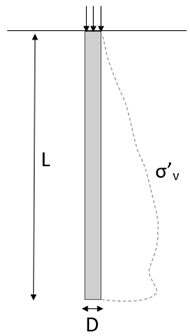Figure 3.1: Single pile problem definition

(Source: Developed by the Researcher)

The problem is developed based on the assessment of the single piles and the L = 16 m, D = 1 m, cu = 140 kPa, and c’ = 15 kPa has been selected. The results are derived, in the context, with the help of the values of stress and strain and the drained and undrained cohesion has been developed. Thus, the relevant results have been developed.

## 3.3 PLAXIS modelling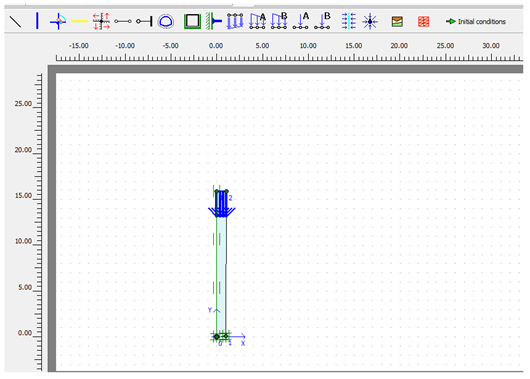Figure 3.2: PLAXIS Design

(Source: Self-created)

The design has been developed based on the system approaches and the Single pile system is effectively implied with the implications of the assessments. These can be relevant to the implications of the system assessments and the following results have been developed that provides effectiveness for configurations (Jostad et al. 2020, p.699). The configurations are useful to derive the relevant data from the system. These designs are hence realized with the development of the beam. Sand is used as the material for primary development. A design is developed according to the design specifications and these are effective in terms of deriving the idea on the system performance.

## 3.4 Results & Discussion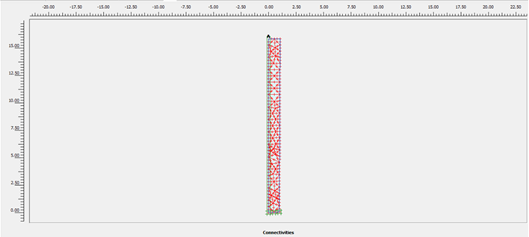Figure 3.3: PLAXIS Output

(Source: Self-created)

The design outputs have been implied and these are required to be analysed with the approaches to system performance. These assessments are subjective in terms of determining a difficult approach for the system (Malekjafarian et al. 2021, p.1015). An effective system is developed based on the materials of construction and these assessments are necessary for better construction of the system. Thus, an effective design needs to be developed for this particular system and the other parameters are also required to be assessed. Thus, an effective system construction is possible and these can be relevant in terms of developing a suitable system and its performance. These can also be relative to the objections and implications of the systems and the rational development of the system is possible.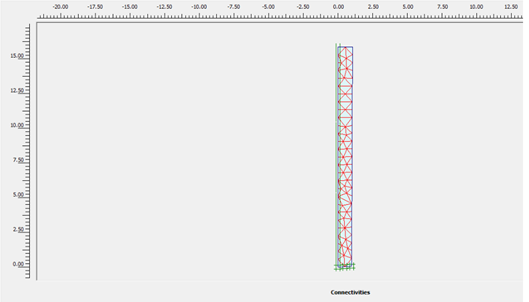Figure 3.4: PLAXIS Output

(Source: Self-created)

The relevance of this modified output can be subjective in terms of relevant development issues and these measures are objective in terms of mitigating the issues. The development is a new system with the axis-symmetric model in PLAXIS 2D (Zhussupbekov et al. 2019, p.144). The designs are hence effective in providing ideas on the system performance. The capability of the system issues is relative in terms of mitigating these issues and this is relevant in terms of assessments.

## 3.5 Conclusion

The assessment has been effective in terms of deriving the ideas on the system parameters like shear stress and normal stress. These are subjective to derive the undrained cohesion and drained cohesion from the angles of the friction and also, the numerical and PLAXIS simulations have been developed. These assessments have been subjected to derive the stress and strain in the system and these are effectively analysed with normal calculations and simulation assessments. The results have described that the system is effective with the development of undrained and drained cohesion. These can be analysed with the implementation of the system performance. The nature and characteristics of the system are effectively assessed and implied. The differential calculations have also been developed from the hand-calculation and PLAXIS simulation. These results have provided a better idea of the system Single pile and these can be subjected to analyse the effectiveness of the results.

# References

Alander, P., Perea-Lowery, L., Vesterinen, K., Suominen, A., SÄilynoja, E. and Vallittu, P.K., 2020. Layer structure and load-bearing properties of fibre reinforced composite beam used in cantilever fixed dental prostheses. Dental Materials Journal, pp.2019-428.

Chanmee, N., DT, B., Hino, T. and LG, L., 2016. Analysis and simulations of erosion protection designs using the PLAXIS 2D and Slide programs. Japanese Geotechnical Society Special Publication2(29), pp.1075-1078.

Jostad, H.P., Dahl, B.M., Page, A., Sivasithamparam, N. and Sturm, H., 2020. Evaluation of soil models for improved design of offshore wind turbine foundations in dense sand. Géotechnique, 70(8), pp.682-699.

Lam, A.K., 2018. An engineering solution for a hillside project in Hong Kong. Geotechnical Research, 5(3), pp.170-181.

Malekjafarian, A., Jalilvand, S., Doherty, P. and Igoe, D., 2021. Foundation damping for monopile supported offshore wind turbines: A review. Marine Structures, 77, p.102937.

Som, N., 2017. Geotechnical Challenges of Kolkata Metro Construction. GEOTECHNICAL ENGINEERING, 48(2), pp.72-79.

Uray, E., Çarba?, S., Erkan, ?.H. and Tan, Ö., 2019. Parametric investigation for discrete optimal design of a cantilever retaining wall. Challenge Journal of Structural Mechanics5(3), pp.108-120.

Yadav, P., Singh, D.K., Dahale, P.P. and Padade, A.H., 2021. Analysis of retaining wall in static and seismic condition with inclusion of geofoam using Plaxis 2D. In Geohazards (pp. 223-240). Springer, Singapore.

YAVAN, O., ONUR, M.?. and TUNCAN, A., 2020.BEHAVIOR OF CANTILEVER RETAINING WALLS UNDER STAT?C AND DYNAMIC LOADS CONSTRUCTED IN SATURATED CLAY SOIL.

Zhao, L. and You, G., 2018. Stability study on the northern batter of MBC Open Pit using Plaxis 3D. Arabian Journal of Geosciences, 11(6), pp.1-11.

Zhussupbekov, A., Omarov, A. and Tanyrbergenova, G., 2019. Design of anchored diaphragm wall for deep excavation. International Journal, 16(58), pp.139-144.

# Appendices

## Appendix B: Additional results and oversized figures (e.g. PLAXIS screen shoots)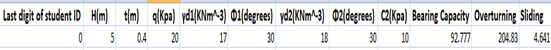Figure 2.2: PLAXIS Design

(Source: Self-created)Figure 2.3: PLAXIS Output

(Source: Self-created)Figure 2.4: PLAXIS Output

(Source: Self-created)Figure 3.2: PLAXIS Design

(Source: Self-created)Figure 3.3: PLAXIS Output

(Source: Self-created)